Next: Thomson Scattering Up: Radiation and Scattering Previous: Antenna Directivity and Effective

# Antenna Arrays

Consider a linear array of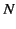half-wave antennas arranged along the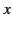-axis with a uniform spacing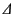. Suppose that each antenna is aligned along the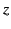-axis, and also that all antennas are driven in phase. Let one end of the array coincide with the origin. The field produced in the radiation zone by the end-most antenna is given by [see Equation (1231)]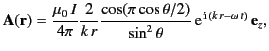(1263)

where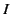is the peak current flowing in each antenna. The fields produced at a given point in the radiation zone by successive elements of the array differ in phase by an amount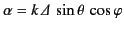. Here,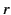,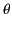,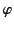are conventional spherical polar coordinates. Thus, the total field is given by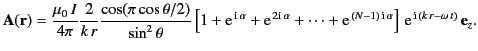(1264)

The series in square brackets is a geometric progression in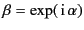, the sum of which takes the value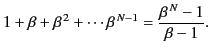(1265)

Thus, the term in square brackets becomes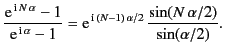(1266)

It follows from Equation (1232) that the radiation pattern due to the array takes the form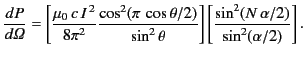(1267)

We can think of this formula as the product of the two factors in large parentheses. The first is just the standard radiation pattern of a half-wave antenna. The second arises from the arrangement of the array. If we retained the same array, but replaced the elements by something other than half-wave antennas, then the first factor would change, but not the second. If we changed the array, but not the elements, then the second factor would change, but the first would remain the same. Thus, the radiation pattern as the product of two independent factors, the element function, and the array function. This independence follows from the Fraunhofer approximation, (1221), which justifies the linear phase shifts of Equation (1222).

The array function in the present case is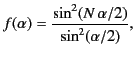(1268)

where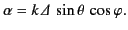(1269)

The function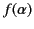has nulls whenever the numerator vanishes: that is, whenever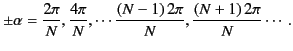(1270)

However, when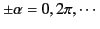, the denominator also vanishes, and the l'Hôpital limit is easily seen to be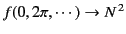. These limits are known as the principal maxima of the function. Secondary maxima occur approximately at the maxima of the numerator: that is, at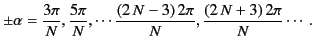(1271)

There are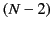secondary maxima between successive principal maxima.

Now, the maximum possible value of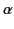is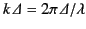. Thus, when the element spacingis less than the wavelength there is only one principal maximum (at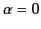), directed perpendicular to the array (i.e., at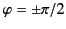). Such a system is called a broadside array. The secondary maxima of the radiation pattern are called side lobes. In the direction perpendicular to the array, all elements contribute in phase, and the intensity is proportional to the square of the sum of the individual amplitudes. Thus, the peak intensity for anelement array is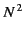times the intensity of a single antenna. The angular half-width of the principle maximum (in) is approximately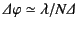. Although the principal lobe clearly gets narrower in the azimuthal angleasincreases, the lobe width in the polar angleis mainly controlled by the element function, and is thus little affected by the number of elements. A radiation pattern which is narrow in one angular dimension, but broad in the other, is called a fan beam.

Arranging a set of antennas in a regular array has the effect of taking the azimuthally symmetric radiation pattern of an individual antenna and concentrating it into some narrow region of azimuthal angle of extent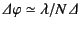. The net result is that the gain of the array is larger than that of an individual antenna by a factor of order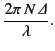(1272)

It is clear that the boost factor is of order the linear extent of the array divided by the wavelength of the emitted radiation. Thus, it is possible to construct a very high gain antenna by arranging a large number of low gain antennas in a regular pattern, and driving them in phase. The optimum spacing between successive elements of the array is of order the wavelength of the radiation.

A linear array of antenna elements that are spaced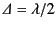apart, and driven with alternating phases, has its principal radiation maximum along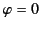and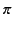, because the field amplitudes now add in phase in the plane of the array. Such a system is called an end-fire array. The direction of the principal maximum can be changed at will by introducing the appropriate phase shift between successive elements of the array. In fact, it is possible to produce a radar beam that sweeps around the horizon, without any mechanical motion of the array, by varying the phase difference between successive elements of the array electronically.Next: Thomson Scattering Up: Radiation and Scattering Previous: Antenna Directivity and Effective
Richard Fitzpatrick 2014-06-27